## A species of animal is discovered on an island. Suppose that the population size P(t) of the species can be modeled by the following functio

Question

A species of animal is discovered on an island. Suppose that the population size P(t) of the species can be modeled by the following function, where time t is measured in years.
P(t) =800/1+ 3e^-0.29t.
Find the initial population size of the species and the population size after 10 years. Round your answers to the nearest whole number as necessary.

in progress 0
2 months 2021-08-18T07:08:41+00:00 1 Answers 1 views 0

The initial population is 200

The population after 10 years is 687

Step-by-step explanation:

Given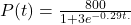Solving (a): The initial population

Here: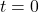Substitute 0 for t in P(t)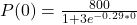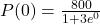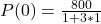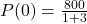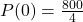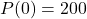The initial population is 200

Solving (b): Population after 10 years.

Here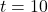Substitute 10 for t in P(t)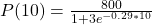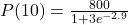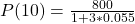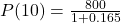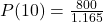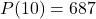— approximated.

The population after 10 years is 687